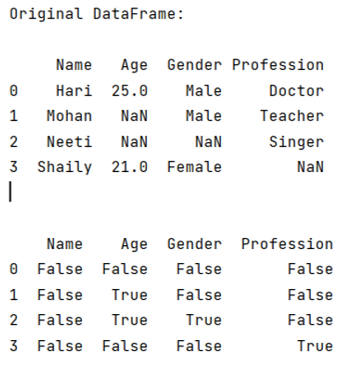# How to select rows with one or more nulls from a Pandas DataFrame without listing columns explicitly?

Given a DataFrame with some null values in some rows, we need to select those null values. By Pranit Sharma Last updated : September 20, 2023

Rows in pandas are the different cell (column) values that are aligned horizontally and also provide uniformity. Each row can have the same or different value. Rows are generally marked with the index number but in pandas we can also assign index names according to the needs. In pandas, we can create, read, update and delete a column or row value.

## Problem statement

Given a DataFrame with some null values in some rows, we need to select those null values.

## Selecting rows with one or more nulls from a Pandas DataFrame without listing columns explicitly

For this purpose, we will use pandas.isnull() method. This method is used to traverse along the entire DataFrame and returns another DataFrame with Boolean values. It returns True if there is some NaN value and False if not.

Note

To work with pandas, we need to import pandas package first, below is the syntax:

```import pandas as pd
```

Let us understand with the help of an example.

## Python program to select rows with one or more nulls from a Pandas DataFrame without listing columns explicitly

```# Importing pandas package
import pandas as pd

# To create NaN values, you must import numpy package,
# then you will use numpy.NaN to create NaN values
import numpy as np

# Create an empty list
result = []

# Creating a dictionary with some NaN values
d={
"Name":['Hari','Mohan','Neeti','Shaily'],
"Age":[25,np.NaN,np.NaN,21],
"Gender":['Male','Male',np.NaN,'Female'],
"Profession":['Doctor','Teacher','Singer',np.NaN]
}

# Now we will create DataFrame
df = pd.DataFrame(d)

# Viewing the original DataFrame
print("Original DataFrame:\n")
print(df,"\n\n")

# Using pd.isnull(df)
print(pd.isnull(df))
```

### Output

The output of the above program is: